## Characteristics of Rate of Rise of Restriking Voltage:

The important Characteristics of Rate of Rise of Restriking Voltage which affect the circuit breaker performance are:

• amplitude factor,
• rate of rise of restriking voltage (RRRV).

Amplitude Factor: The amplitude factor is defined as the ratio of the peak of transient voltage to the peak system frequency voltage. BS 116 specify the standard for evaluating amplitude factor.

Rate of Rise of Restriking Voltage (RRRV): The RRRV is defined as the slope of the steepest tangent to the restriking voltage curve. It is expressed in volts per microsecond. For a restriking voltage having a single frequency transient component the RRRV is obtained by dividing the maximum amplitude of the oscillation by the duration of the first half wave. Higher values of natural frequencies can be related with higher rates of Characteristics of Rate of Rise of Restriking Voltage. It is clear that other things being equal, the duty of a circuit breaker is much more severe when used in a-network of high natural frequency, than on a. network having low natural frequency because the average RRRV is very much greater in the first case. In the latter case the voltage across the contacts of the circuit breaker rises slowly thereby giving longer time for building up of the dielectric strength.

### Expression for RRRV:

The expression for the restriking voltage has already been derived in Eq. (14.2).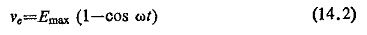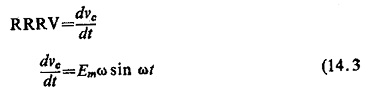Maximum value of RRRV occurs when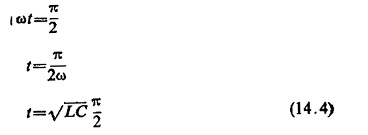Hence maximum value of RRRV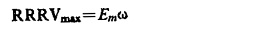Further, the peak restriking voltage occurs when vc is maximum, i.e. at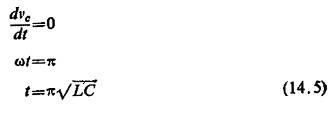### Factors Affecting the Restriking Voltage Characteristics:

After current zero, the initial rate of rise and the peak value of the restriking voltage stressing the contact gap depend on the configuration of the network, its natural frequency and on the relative position of the resistances (in parallel or series with the main capacitance of the circuit) as shown in Fig. (14.11). It is quite logical to expect that the presence of resistance damps the Characteristics of Rate of Rise of Restriking Voltage, The true nature of attenuation is complicated since the losses depend on many things such as conductor resistance, iron loss, dielectric loss, corona, etc. These factors vary with frequency and voltage in different ways.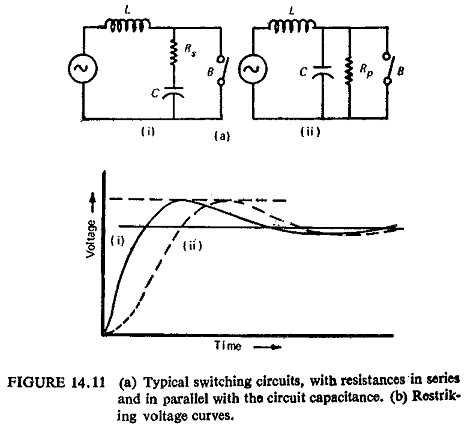In a network consisting of generators, transformers, reactors, and transmission line, each of them exerts its own damping. Usually the attenuation due to them is too small to be relied upon for improvement in the performance of a breaker. Where high RRRV are expected circuit breakers with shunt resistances (Fig. (14.11)) are used. Now to ensure that the voltage across the breaker builds up exponentially to the 50 Hz recovery voltage, without overshoot, instead of exhibiting the oscillatory doubling effect associated with an undamped circuit, the value of resistance Rp needed to achieve critical damping is 1/2 √L/C. Figure (14.12) shows the restriking voltage critically damped and other related wave shapes.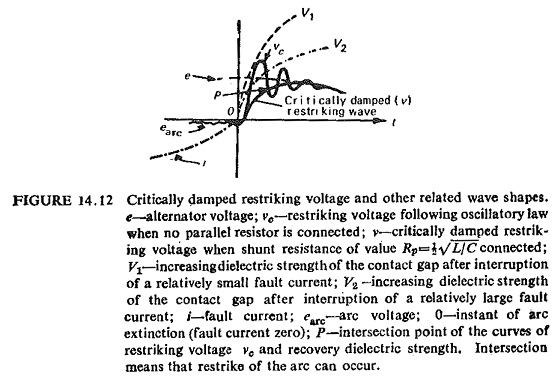It can thus be inferred that the shunt resistance across the breaker modifies the oscillatory restriking voltage into an aperiodic wave (curve V). This entails the arc to be extinguished even though the dielectric strength of the gap increases only relatively slowly (curve V2) as a result of severe short circuit. Inclusion of the shunt resistor thus increases the rupturing capacity of the breaker.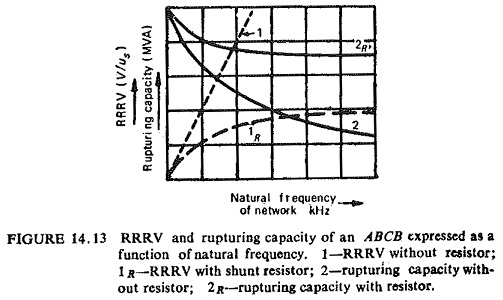Figure (14.13) shows the relation of RRRV and rupturing capacity of an air-blast circuit breaker with and without shunt resistors as a function of natural frequency. It has already been pointed out earlier that without shunt resistance the RRRV is directly proportional to the natural frequency of the circuit, and the rupturing capacity of the breaker therefore falls rapidly with increasing frequencies. In the case of breaker with shunt resistance the RRRV cannot exceed a certain value determined by the resistor and hence the rupturing capacity does not fall to that extent. For higher values of natural frequencies the advantage gained in the rupturing capacity is more.

Scroll to Top